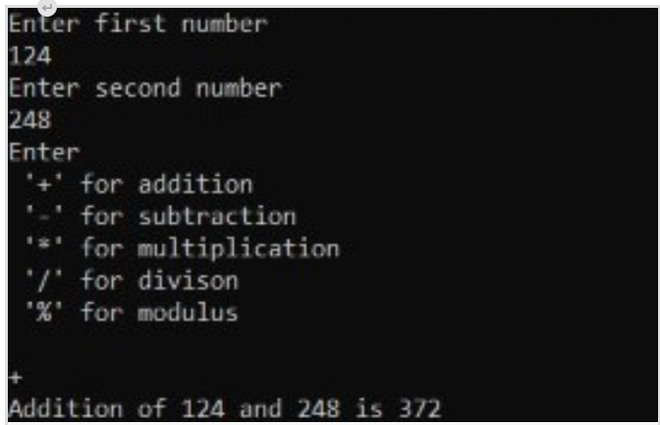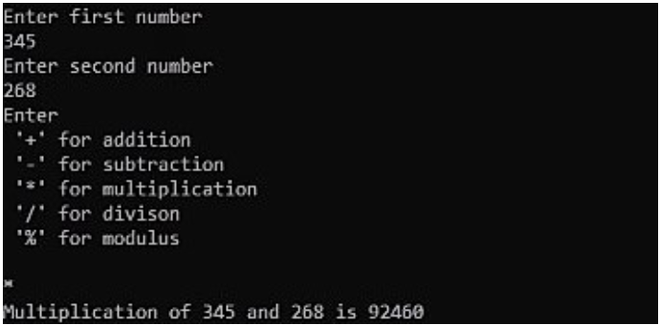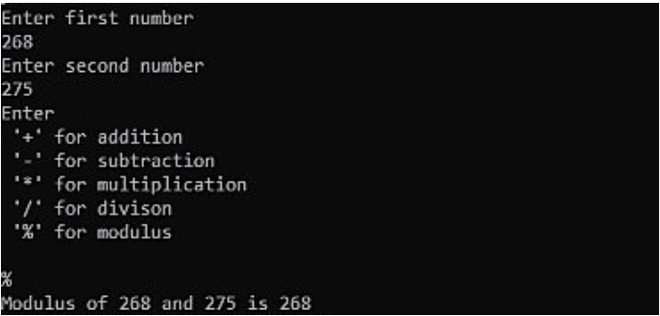# TCL script to perform appropriate arithmetic operations using switch statement

In this article, we will cover the overview of TCL script to perform appropriate arithmetic operations using the switch statement and will implement with the help of an example. Let’s discuss it one by one.

Pre-requisite –

Overview :
We will understand the basic syntax of a switch statement in Tool Command Language, by a simple example. In this example, our objective is to perform an arithmetic operation based on the symbol we specify. For example, if we specify ‘+’ then addition must be performed and like-wise.

Steps to perform appropriate arithmetic operations using the switch statement :
Here, we will implement TCL script to perform appropriate arithmetic operations using the switch statement step by step as follows.

Step-1: Input numbers :
Firstly, we need to read two numbers a and b.

```puts "Enter the first number "
gets stdin a
puts "Enter the second number "
gets stdin b```

Secondly, we need to prompt to enter the desired character and have to read it.

```puts "Enter \n '+' for addition\n '-' for subtraction\n '*' for multiplication\n
'/' for division"
gets stdin ch```

Step-3: Writing Switch Statement :
Now, we need to write a switch statement, to map to the appropriate symbol.

```switch \$ch {
"+" {
set result [expr \$a+\$b]
puts "Addition of \$a and \$b is \$result"
}
"-" {
set result [expr \$a-\$b]
puts "Subtraction of \$a and \$b is \$result"
}
"*" {
set result [expr \$a*\$b]
puts "Multiplication of \$a and \$b is \$result"
}
"/" {
set result [expr \$a*\$b]
puts "Division of \$a and \$b is \$result"
}
"%" {
set result [expr \$a%\$b]
puts "Modulo of \$a and \$b is \$result"
}
default {
puts "Enter the appropriate input"
}
}```

Note –
The syntax for the switch statement should be exactly as shown above. If you neglect the spaces or type the opening curly brace in a new line, the result will be an error. And of course, we only use double quotes for characters in TCL.

Step-4: Understanding the syntax :
Now to understand the syntax better, the C programming version of the addition (+) case is shown below.

```case '+':
result=a+b;
printf("Addition of %d and %d is %d",a,b,result);
break;```

As you can see, we don’t use the keyword case followed by a colon ‘:‘ in TCL. Instead, we directly specify the required action statement, followed by the body in the curly braces. The break statement is not necessary for the TCL script.

Step-5: TCL script to perform appropriate arithmetic operations using the switch statement :
Now, let us look at how the complete code using TCL would like as follows.

Code –

```puts "Enter the first number "
gets stdin a
puts "Enter the second number "
gets stdin b
puts "Enter\n '+' for addition\n '-' for subtraction\n '*' for multiplication\n '/' for division"
gets stdin ch
switch \$ch {
"+" {
set result [expr \$a+\$b]
puts "Addition of \$a and \$b is \$result"
}
"-" {
set result [expr \$a-\$b]
puts "Subtraction of \$a and \$b is \$result"
}
"*" {
set result [expr \$a*\$b]
puts "Multiplication of \$a and \$b is \$result"
}
"/" {
set result [expr \$a*\$b]
puts "Division of \$a and \$b is \$result"
}
"%" {
set result [expr \$a%\$b]
puts "Modulo of \$a and \$b is \$result"
}
default {
puts "Enter the appropriate input"
}
}```

Output :
The outputs for a set of inputs are as follows.For Multiplication –For modulus –Unlock the Power of Placement Preparation!
Feeling lost in OS, DBMS, CN, SQL, and DSA chaos? Our Complete Interview Preparation Course is the ultimate guide to conquer placements. Trusted by over 100,000+ geeks, this course is your roadmap to interview triumph.